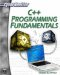# While Loops

While loops are almost identical to do loops. They are simply structured a little bit differently. The do loop has the condition to be evaluated at the end of the loop, whereas the while loop has it at the beginning.

`while(condition) { }`

The following is an example of a while loop.

### Example 5.6

Step 1: Type the following code into your favorite text editor.

`#include <iostream> using namespace std; int main() {  int i = 0;  while(i < 10)  {    cout << "I is " << i << "\n";    i++;  }  return 0; }// end of main`

Step 2: Compile the code.

Step 3: Run the code. You should see something like the image in Figure 5.5.Figure 5.5: While loops.

As you can see, all loop structures accomplish essentially the same goal. They execute a given block of code a certain number of times. The only differences are where you check the value of the loop to see if you will continue looping, and where you increment the loop counter. C++ loops are best described by the old axiom ”There is more than one way to skin a cat.”C++ Programming Fundamentals (Cyberrookies)
ISBN: 1584502371
EAN: 2147483647
Year: 2005
Pages: 197
Authors: Chuck Easttom